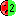# Selenium

Selenium was founded in 1958 in the city of Borges (Brazil). After two years the first tweeters were produced. This was followed by loudspeakers for the living area. In the 80's loudspeakers for cars were produced, followed by loudspeakers for professional sound reinforcement (PA).

## Compression driver for Horns + Tweeter## Selenium D3505Ti-Nd DPD

order no. sel-28031046

EUR 384,07excl. VAT: € 322.75 / \$ 387.38

2 inch high frequency compression driver with titanium cone.

• power handling (continuous/programme) = 150 W
• frequency range = 500-20000 Hz
• impedance R = 16 Ohm
• sound pressure level SPL = 111 dB (2,83V; 1m)
• DC resistance Re = 8,5 Ohm
• voice coil diameter = 75 mm
• overall diameter d = 126 mm
• mounting depth (not countersunk) t = 71 mm
• mass m = 2,8 kg
PDF data sheet

## Selenium D305

order no. sel-28031005

EUR 149,14excl. VAT: € 125.33 / \$ 150.43

.

• power handling (continuous/programme) = 100/150 W
• frequency range = 400-9000 Hz
• impedance R = 8 Ohm
• sound pressure level SPL = 110 dB (2,83V; 1m)
• DC resistance Re = 6 Ohm
• voice coil diameter = 75 mm
• overall diameter d = 169 mm
• mounting depth (not countersunk) t = 76 mm
• mass m = 5 kg
PDF data sheet

## Selenium D405

order no. sel-28031006

EUR 232,00excl. VAT: € 194.96 / \$ 234.00

.

• power handling (continuous/programme) = 150/200 W
• frequency range = 300-7000 Hz
• impedance R = 8 Ohm
• sound pressure level SPL = 110 dB (2,83V; 1m)
• DC resistance Re = 6 Ohm
• voice coil diameter = 100 mm
• overall diameter d = 205 mm
• mounting depth (not countersunk) t = 86 mm
• mass m = 8,3 kg
PDF data sheet

## Selenium D4400Ti

order no. sel-28031016

EUR 388,94excl. VAT: € 326.84 / \$ 392.29

2 inch high frequency compression driver with titanium cone.

• power handling (continuous/programme) = 200/250 W
• frequency range = 400-20000 Hz
• impedance R = 8 Ohm
• sound pressure level SPL = 112 dB (2,83V; 1m)
• DC resistance Re = 4,8 Ohm
• voice coil diameter = 100 mm
• overall diameter d = 230 mm
• mounting depth (not countersunk) t = 163 mm
• mass m = 10,2 kg
PDF data sheet

## Horn## Selenium HC23-25

order no. sel-28056014

EUR 15,01excl. VAT: € 12.61 / \$ 15.14

Horn for a compression driver with 1 inch throat.

• Throat diameter = 1 inch
• nominal angle (HxV) = 100x40 Deg
• Cut off frequency : 600 Hz
• over all dimensions (HxWxD) = 128x254x145 mm
• net weight = 0,34 kg
PDF data sheet

## Selenium HM11-25

order no. sel-28056033

EUR 9,16excl. VAT: € 7.70 / \$ 9.24

Horn for a compression driver with 1 inch throat.

• Throat diameter = 1 inch
• nominal angle (HxV) = 60x60 Deg
• Cut off frequency : 1200 Hz
• over all dimensions (HxWxD) = 115x115x75 mm
• net weight = 0,1 kg
PDF data sheet

## Tweeter## Selenium DT150

order no. sel-28058002

EUR 42,18excl. VAT: € 35.45 / \$ 42.54

.

• power handling (continuous/programme) = 80/150 W
• frequency range = 1500-15000 Hz
• impedance R = 8 Ohm
• sound pressure level SPL = 108 dB (2,83V; 1m)
• DC resistance Re = 8 Ohm
• voice coil diameter = 25 mm
• overall diameter d = 115 mm
• mounting depth (not countersunk) t = 120 mm
• mass m = 0,8 kg
PDF data sheet

## Selenium ST350

order no. sel-28003017- not available

42 mm tweeter with high quality cone.

• power handling (continuous/programme) = 150/200 W
• frequency range = 2500-20000 Hz
• impedance R = 8 Ohm
• sound pressure level SPL = 111 dB (2,83V; 1m)
• DC resistance Re = 6,2 Ohm
• voice coil diameter = 42 mm
• mounting diameter d = 104 mm
• overall diameter d = 130 mm
• mounting depth (not countersunk) t = 98 mm
• mass m = 1,42 kg
PDF data sheet

## Selenium ST400

order no. sel-75003029

EUR 78,96excl. VAT: € 66.35 / \$ 79.64

42 mm tweeter with high quality cone.

• power handling (continuous/programme) = 200/400 W
• frequency range = 4000-20000 Hz
• impedance R = 8 Ohm
• sound pressure level SPL = 111 dB (2,83V; 1m)
• DC resistance Re = 6,2 Ohm
• voice coil diameter = 42 mm
• mounting diameter d = 103 mm
• overall diameter d = 140 mm
• mounting depth (not countersunk) t = 87 mm
• mass m = 1,69 kg
PDF data sheet

The cabinet volume calculations are executed considering an external resistance of 0.3 Ohm. We are using, if no other data is known or available, the information supplied by the manufacturer.

Please note: the frequency response doesn't say much about the cut-off frequency inside the cabinet! Therefore, we mostly give you 2 examples with dimensioning considerations and an indication, how low the loudspeaker will reproduce.

Home